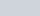ChemTalk

# Heat of Fusion Explained## Core Concepts

In this article, you will learn about heat of fusion, including its thermodynamics and its applications. After this article, you will be able to understand the nature of heat of fusion as well as use it to solve thermochemistry problems.

## What is Heat of Fusion?

Heat of fusion, also called enthalpy of fusion or latent heat of fusion, is a quantity of energy needed to melt or freeze a substance under conditions of constant pressure. When studying chemistry, “fusion” simply has the same definition as melting. In the classroom, you mostly use heat of fusion when a substance is at its melting point or freezing point. In such cases, most think of heat of fusion as a constant.

For instance, water has a heat of fusion of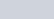at its melting point of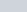. This means that, at, one gram of liquid water must release 334 Joules of energy to completely freeze into ice. Also, one gram of ice must absorb 334 Joules of energy to completely melt atYou can calculate the amount of heat energy needed to change a substance’s phase at its melting point using the following heat of fusion equation: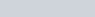•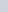: Total change in heat energy (in Joules)
•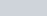: Heat of fusion of substance (in Joules per gram)
•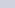: Mass of substance (in grams)

#### Molar Heat of Fusion

If you know the molar mass of the substance, you can easily convert it into a molar heat of fusion. Water has a molar mass of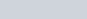, so its molar heat of fusion would be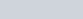(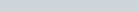). Consequently, to calculate the total change in energy, you would instead have to use moles instead of mass: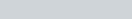•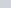: Moles of substance

Heat of fusion has interested chemists and physicists because it suggests that a substance can absorb or release energy without changing temperature. Indeed, when one mole of ice atabsorbs 6020 Joules of heat, the resulting liquid water will also have a temperature of. To understand the peculiarity of this phenomenon, we first need to talk about energy changes that don’t involve a phase change.

## How do substances usually change in temperature?

Typically, when a substance absorbs or releases heat energy, its temperature then changes in response. The amount of temperature change is governed by the substance’s specific heat, which is a quality intrinsic to a substance and does not depend on how much of the substance you have. The following equation details the relationship between heat energy, specific heat, and temperature.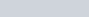•: Change in heat (in Joules)
•: Mass of the substance (in grams)
•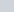: Specific heat of substance (in Joules per gram per degree Celsius)
•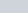: Change in Temperature (in degrees Celsius)

In this way, you can think of specific heat as the amount of energy needed to change one gram of a substance’s temperature by one degree Celsius. For instance, the specific heat of gold is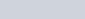. This means that one gram of pure gold heats by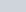when it absorbs 0.128 Joules of energy. Conversely, when 0.128 Joules of energy are extracted from the gold, its temperature lowers by. To learn more about how to use specific heat, check out this article.

Since temperature and heat have a directly proportional relationship, a heat versus temperature graph of a substance without phase changes is linear. When the graph includes phase changes, a strange-looking piecewise slope emerges with flat stretches that correspond to melting and vaporization. As a side note, the phase change between gas and liquid is governed by a “heat of vaporization” that functions identically to heat of fusion.

So, given that temperature and heat energy have a direct relationship usually, why does temperature not change during phase transitions? To understand why, we need to investigate the thermodynamics of phase transitions.

## The Thermodynamics behind Heat of Fusion

#### Temperature and Internal Energy

First, we need to talk about what “temperature” really means. Chemists and physicists define temperature as the average kinetic energy per molecule of a substance. Kinetic energy depends on the mass and speed of a particle. Because heating a substance does not involve changes in molecular mass, only the speed of the molecules changes. Thus, when a substance absorbs heat energy, its molecules then move faster, indicating an increased temperature.

Importantly, a system’s temperature is proportional to a quantity called the internal energy of the system. Thus, any changes to a system’s temperature will involve a similar change in its internal energy.

The first law of thermodynamics states that any change in the internal energy of a system (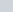) equals the sum of heat given off or absorbed and work done by or on the system: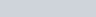•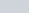: Internal energy; positive for increasing temperature, negative for decreasing temperature (in Joules)
•: Heat; positive for heat absorbed, negative for heat released (in Joules)
•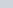: Work; positive for work done on the system, negative for work done by the system (in Joules

#### Work

Generally, when dealing with some substance in a vessel, the only work that the substance can perform is expansion or compression work. This involves the substance changing volume with or against an external pressure. However, only gases can perform substantial expansion or compression, and heat acts oppositely to maintain the gas’s internal energy. For instance, when heating a gas (positive heat), the gas will expand (negative work), resulting in no change in internal energy. Further, when compressing a gas (positive work), the gas will release heat energy (negative heat).

Unlike gases, liquids and solids don’t change much in volume when heated or cooled. When a liquid or solid is heated or cooled, virtually no work is performed. Thus, any heat absorbed increases the substance’s internal energy, raising its temperature.

Liquids and solids do experience nonzero work, however, when changing phases. This is because molecules are often closer together in solid form than in liquid form. As a result, a solid melting into a liquid must perform expansion, and a liquid must compress to solidify.

This phenomenon precisely explains the nature of the heat of fusion. Once a solid is heated to its melting point, any further heat energy inputted into the solid is used to expand it into a liquid. Thus, the heat and work of the substance counteract each other, resulting in no change in internal energy, allowing the substance to stay at its melting point until it becomes entirely liquid.Heating curve of a substance for all three phases. Phase change lines indicate heat exchange without temperature change.

## Heat of Fusion Practice Problems

Problem 1

How much energy does it take to convert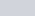of ice at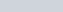into water at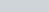? The specific heat of ice is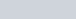and the specific heat of liquid water is.

Problem 2

Rather than melt into liquid, solid CO2 (also known as dry ice) sublimates into gas under atmospheric pressure at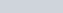. Appropriately, dry ice has a heat of sublimation of.

You have a chemical reaction that will give off approximately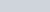, but you want to make sure it doesn’t get warmer than. What is the minimum number of grams of dry ice should you surround the reaction flask to keep it cold? (Hint: you’ll want enough dry ice to absorb all the heat through sublimation so that no heat is available to warm up the reaction).

## Heat of Fusion Practice Problem Solutions

1:2: